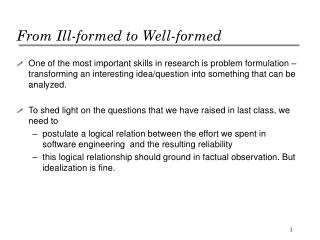DownloadDownload PresentationFrom Ill-formed to Well-formed

# From Ill-formed to Well-formed

Download Presentation## From Ill-formed to Well-formed

- - - - - - - - - - - - - - - - - - - - - - - - - - - E N D - - - - - - - - - - - - - - - - - - - - - - - - - - -
##### Presentation Transcript

1. From Ill-formed to Well-formed • One of the most important skills in research is problem formulation – transforming an interesting idea/question into something that can be analyzed. • To shed light on the questions that we have raised in last class, we need to • postulate a logical relation between the effort we spent in software engineering and the resulting reliability • this logical relationship should ground in factual observation. But idealization is fine.

2. Assumptions Grounded in Observations • 1) The more complex the software project is, the harder it is to make it reliable. For a given degree of complexity, the more effort that we can devote to software engineering, the higher the reliability. • 2) The obvious errors are spotted and corrected early during the development. As time passes by, the remaining errors are subtler, more difficult to detect and correct. • 3) There is only a finite amount of effort (budget) that we can spend on any project.

3. C=1 C = 2 Figure 1: Reliability and Complexity From Assumptions to Model • These observations suggest that, for a normalized mission duration t = 1, the reliability of a software system can be expressed as an exponential function of the software complexity, C, and available development effort, E, in the form of R(E, C) =e-C /E. As we can see, R(E, C) rises as effect E increases and decreases as complexity C increases. There is, however, another way to model it.

4. Analysis • For 3-version programming: • the reliability of each version when efforts are equally allocated is R = exp( – c/(E/3)) • The system works if all of 3 works or any 2 out 3 works. Thus, the reliability of the system Rs = R3 + 3(R2*(1-R)). Note that this analysis assumes faults in different versions are independent, a favorable assumption. • For recovery block with a perfect acceptance test (favorable assumption), if any version works the system works. • For the case of 3 alternatives without complexity reduction and with equal effort allocation, we have R = exp( –c/(E/3)) and Rs = 1 – (1 – R)3 • if you divide the effort equally among 2 alternatives but one has only 0.5c complexity, then R1 = exp(–c/(E/2)) and R2 = exp(–0.5c/(E/2)) and system reliability Rs = 1 – (1 – R1)(1–R2). • You can try out different effort allocation methods and different complexity reductions and see the results (plot them and try to find a qualitative pattern).

5. Single version programming 3-version programming Figure 2: Effect of Divided Efforts in 3-version Programming From Assumption to Model - 3 • Single version vs 3 version (equal allocation)

6. RB Single version programming RB: Recovery Block Figure 3: Effect of Dividing Effort in Recovery Block Single version vs Recovery Block • Single version vs Recovery Block (3 alternatives, equal allocation, no complexity reduction)

7. RB2 RB10 RB3 Figure 4: Degree of Diversity Degree of Diversity • RBn, where n is the number of alternatives. (n-way equal allocation, no complexity reduction) Adding diversity to system is kind of liking adding salt to a bowl of soup. A little improves the taste. Too much is counter-productive.

8. RB2L10 RB2 RB2L2 Single version programming Figure 5: Effect of Complexity Reduction Keep it Simple, Stupid! • RB2Ln, where n is the complexity reduction in the alternative to the primary with full functionality. (2-way equal effort allocation, n times complexity reduction in the simple alternative)

9. Using Simplicity to Control Complexity • Ok, simplicity leads to reliability. But we want fancy features that require complex software. Worse, most applications do not have high coverage acceptance tests. • The solution is to use simplicity to control complexity. • use a simple and reliable core that provides the essential service. • Ensure that the reliable core will not compromised by the faults in the bells-and-whistles. • Leverage the reliable core to ensure the overall system integrity in spite of faults in the complex features, even WHEN THERE IS NO EFFECTIVE ACCEPTANCE TESTS.

10. Homework for Next Lecture • In next lecture, we will investigate the idea of forward recovery that embodies the idea of using simplicity to control complexity. • Each team shall give 1 single slide that gives an example in everyday life, other fields of engineering or CS that embodies the idea of using simplicity to control complexity. • Think and think creatively • The class presentation will go on after the mini-lecture.